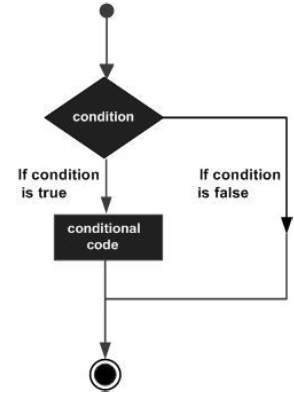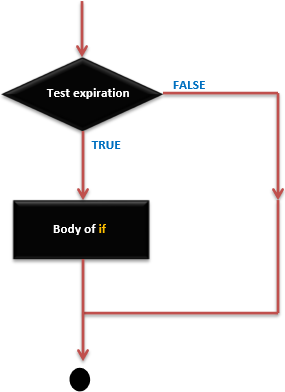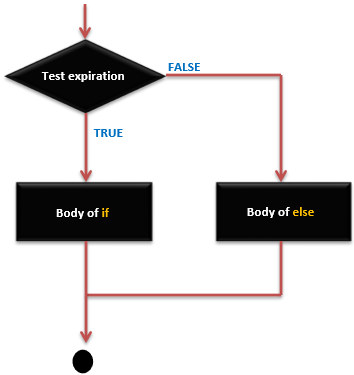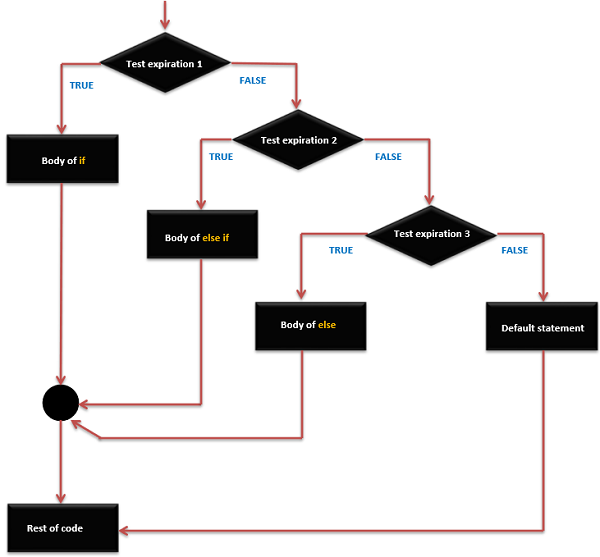# Arduino - 控制语句1. if 语句
2. if...else 语句
3. if...else if...else 语句
4. switch case 语句
5. :? 条件运算符-三元运算符

## if 语句

### 不同形式的 if 语句

if (expression)
statement;


if (expression) {
Block of statements;
}


### if 语句 - 执行顺序### 举例

/* Global variable definition */
int A = 5 ;
int B = 9 ;

Void setup () {

}

Void loop () {
/* check the boolean condition */
if (A > B) /* if condition is true then execute the following statement*/
A++;
/* check the boolean condition */
If ( ( A < B ) && ( B != 0 )) /* if condition is true then execute the following statement*/ {
A += B;
B--;
}
}


## if...else 语句

### if … else 语句语法

if (expression) {
Block of statements;
}
else {
Block of statements;
}


## if … else 语句 - 执行顺序### 举例

/* Global variable definition */
int A = 5 ;
int B = 9 ;

Void setup () {

}

Void loop () {
/* check the boolean condition */
if (A > B) /* if condition is true then execute the following statement*/ {
A++;
}else {
B -= A;
}
}


## if...else if...else 语句

if 语句可以跟着一个可选 else if 语句，这是对有多个条件分支时非常的有用.

• 一个 if 可以有零个或一个 else 语句，它必须在任何其他 if 之后。
• 一个 if 能有 0 到多个 else if 语句，他们必须在 else 之前。
• 一个 else iftrue 后，其余的 else ifelse 语句都不会被检测。

### if … else if … else 语句语法

if (expression_1) {
Block of statements;
}

else if(expression_2) {
Block of statements;
}
.
.
.

else {
Block of statements;
}


## if … else if else else 语句执行顺序### 例

/* Global variable definition */
int A = 5 ;
int B = 9 ;
int c = 15;

Void setup () {

}

Void loop () {
/* check the boolean condition */
if (A > B) /* if condition is true then execute the following statement*/ {
A++;
}
/* check the boolean condition */
else if ((A == B )||( B < c) ) /* if condition is true then
execute the following statement*/ {
C = B* A;
}else
c++;
}


## switch case 语句

break 关键字使得 switch 语句退出，它经常在每个 case 的结尾使用。如果没有 break 语句，switch 语句将继续执行以下表达式直到 break 出现，或者到达 switch 语句的结尾。

### switch case 语句语法

switch (variable) {
case label:
// statements
break;
}

case label: {
// statements
break;
}

default: {
// statements
break;
}


### switch case 语句执行顺序### 举例

switch (phase) {
case 0: Lo(); break;
case 1: Mid(); break;
case 2: Hi(); break;
default: Message("Invalid state!");
}


## ?: 三元条件运算符

### ?: 条件运算符语法

expression1 ? expression2 : expression3


### 举例

/* Find max(a, b): */
max = ( a > b ) ? a : b;
/* Convert small letter to capital: */
/* (no parentheses are actually necessary) */
c = ( c >= 'a' && c <= 'z' ) ? ( c - 32 ) : c;


## 条件算子规则

• expression1 必须是标量表达式; expression2expression3 必须遵守以下规则之一。
• expression2expression3 都必须是算术类型。
• expression2expression3 的结果类型确定。